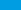ACT Math WorksheetsLooking for FREE printable ACT Math worksheets? Here’s a collection of PDF worksheets for all ACT Math topics.

Need great ACT Math worksheets to help your students prepare for the ACT Mathematics test? If so, then you are in the right place. Here is a comprehensive and perfect collection of FREE ACT Mathematics worksheets that would help students in ACT Math preparation and practice.

Download our free Mathematics worksheets for the ACT test.

Hope you enjoy!

Fractions and Decimals

Logarithms